# Python Program to Add Two Matrices

In this tutorial you will learn about the Python Program to Add Two Matrices and its application with practical example.

In this tutorial, we will learn to create a Python Program to Add Two Matrices using python Programming.

• ## Prerequisites

Before starting with this tutorial we assume that you are best aware of the following Python programming topics:

• Python Operator.
• Basic Input and Output
• Basic Python  programming.
• Python Data type.
• Python in-built Functions.
• Loops in Python.
• Built in Modulus.

A matrix can only be added ,if the  both the matrices have the same dimensions.

for example:-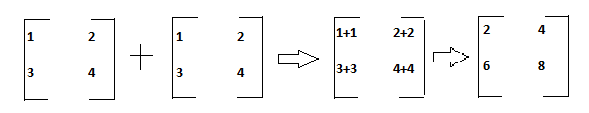To add two matrices of 2*2 we need just to add the corresponding entries means( First element of matrix added to first element of second matrix), and place this sum in the corresponding position in the results. matrix and this process continues till we reach to last elements.

## Python Program to Add Two Matrices

In this program we will add two Matrices using nested for loop . We would first declared and initialized the required variables. Next, we would prompt user to input the values  later we will add two matrices.

## Output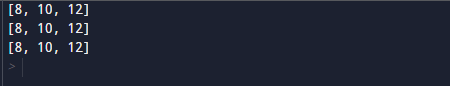In our program above  we going to use nested “for” loops to iterate through each and every rows and columns. within the loop we “add” the corresponding elements of the two matrices and store the result in variable “sum”.

In the above program, we have first declared and initialized a set variables required in the program.

• A = it will hold first matrix
• B= it will hold second matrix
• sum=it will hold added values of matrices

First Matrix.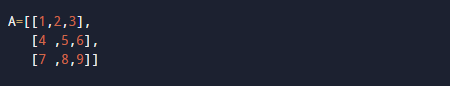Second matrix.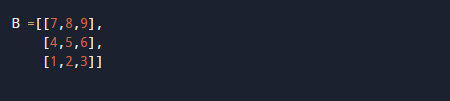and variable sum is initiate to zero.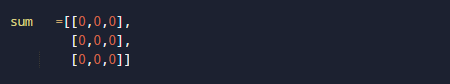Now , we will add two matrices using Nested loop and after that we display it.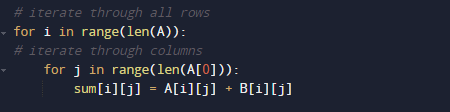Here we use two for loops to iterate through each row and column. We added the corresponding elements of  two matrices and store the result  in the variable “sum” .At last we will display the value stored in variable “sum” after addition of two matrices.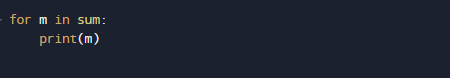Using for loop we displayed the resultant of added matrices.In this tutorial we have learn about the Python Program to Add Two Matrices and its application with practical example. I hope you will like this tutorial.